# Maths Brain Teasers 49:: Complete the Number Series???

in STEMGeeks2 months ago

Hey All; @StemGeeks Mathematician;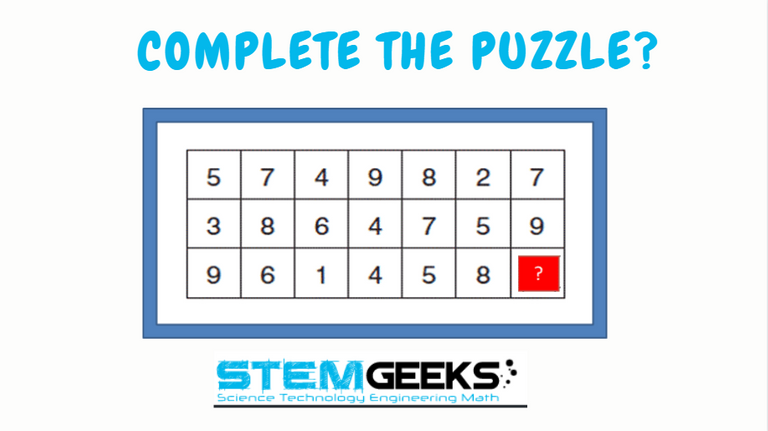Do you love solving puzzles? Then I guess this one is for you.. All that I can assure you is that this is a simple rather very simple puzzle to solve.. No complex mathematics involved at all.. Have a close look at the image given and see if you find; what should replace the question mark? There is logic to resolve the puzzle; try finding out the logic and it should be resolved in minutes.. Guesswork isn't going to help...

With that, I'll leave you all with the Maths Brain Teasers 49:: Complete the Number Series? - Good luck solving iT...

##### Maths Brain Teasers 48:: Can You Solve The Puzzle? - Solved with Explanation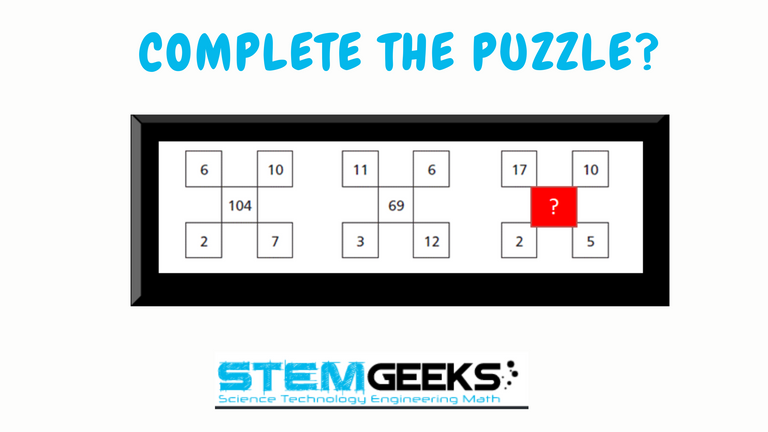Let's have a look at the Step by Step instructions to Solve the Maths Brain Teasers 48 :: Can You Solve The Puzzle???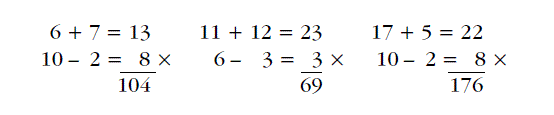• Step 1 First row First Number i.e. 6 is Added by Second Row Secon Number i.e. 7

### 6 + 7 = 13

• Step 2 First Row Second Number i.e. 10 is Subtracted by Second Row First Number i.e. 2

### 10 - 2 = 8

• Step 3 Resultant of both Steps 1 & 2 is Multiplied i.e.

### 13 * 8 = 104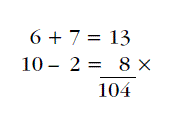In a similar fashion, we are going to solve the Puzzle in Question::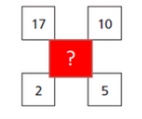• Step 1 First row First Number i.e. 17 is Added by Second Row Secon Number i.e. 5

### 17 + 5 = 22

• Step 2 First Row Second Number i.e. 10 is Subtracted by Second Row First Number i.e. 2

### 10 - 2 = 8

• Step 3 Resultant of both Steps 1 & 2 is Multiplied i.e.

### 22 * 8 = 176

Hence the Answer to Maths Brain Teasers 48:: Can You Solve The Puzzle = 176

### STEM token GiveAway

I'll be again doing a giveaway of STEM tokens to the lucky random winner with the correct answer. For the last contest, which was Maths Brain Teasers 48:: Can You Solve The Puzzle?.

We had 2 entries and I happy to see the engagement by everyone. At the same time, its great to see the detailed answers provided by everyone as to how they arrived at the solution of the puzzle.. Well done guys.. Way to GO... Now the results part; both the entries provided the right answer. And hence; I'm going to split the reward between @chrisparis && @harpreetjanda

Congratulations Guys.. You both WIN yourself 5 STEM tokens. You should be having the rewards in your STEM Wallet Soon.

### Math Quote for the Day::

Here is the motivation to solve the Maths Equation Puzzle?If you like my work, then please spread the Word.. that we do have the Math Brain Teasers competition here @StemGeeks platform. Reblog is much appreciated.

Best RegardsFind Me on the Other Social Media Platforms::PS:: All the Maths Brain Teasers; are made by me using the Pro Canva License Version

Sort:

sum of first 2 numbers (rows) in a column + 1 is number in 3rd row
If sum is a double digit number just use the 2nd digit
5 + 3 + 1 = 9
7 + 8 + 1 = 16 so answer is 6

Last column
7 + 9 + 1 = 17 so answer is 7

Line 1 total 42
Line 2 total 42
Line 3 total 33 + 9 = 42